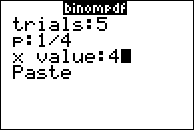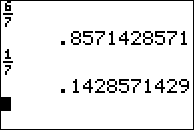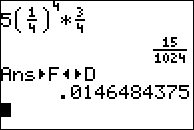•##### Download

•• ##### Device
• TI-83 Plus Family
• TI-84 Plus
• TI-84 Plus Silver Edition
•TI-84 Plus C Silver Edition
•TI-84 Plus CE
• ##### Report an Issue

Precalculus: Binomial Probability in Baseball
by Texas Instruments#### Overview

In this activity, students will explore the link between Pascal's Triangle, the Binomial Theorem, and Binomial Probability Experiments.

#### Key Steps

•Students will determine the probability that a baseball team will win and lose.

•Students will find the binomial expansion of (p + q)5 using Pascal’s Triangle.

•Students will use the graphing calculator to calculate the binomial probability.